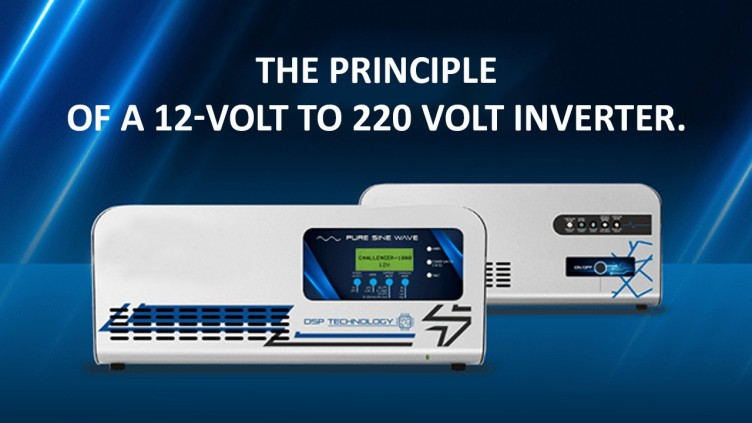Semco university – All about the Lithium-Ion Batteries# Circuit analysis: The principle of a 12 volt to 220 volt inverterThis article will tell you the principle of a 12 Volt to 220 Volt inverter. The schematic diagram is as follows. It looks very simple. Only two triodes and two resistors are used, plus a transformer can generate alternating current at the output end, which can drive 220 V incandescent lamps. It is precisely because the schematic is simple and this circuit is very suitable for beginners, and it is easy to understand what the inverter is like.

Let’s not say that for now, assuming that the power supply has just been turned on at this time, for the moment of power, for the triode Q1, this collector, that is, point A in the schematic diagram is connected to the positive electrode of the power supply. For point B, that is, the base pole is connected to the positive electrode of the power supply by a large power supply, so it satisfies the requirements of the triode conduction. Conditions.

Let’s analyze the triode Q2 again. When the power supply is turned on, its collector, that is, point C is connected to the positive electrode of the power supply, and the base pole is also point D, which is connected to the positive electrode of the power supply through a large power resistance, which also meets the on-through conditions.

Therefore, at the moment the power supply is turned on, the state of the triode Q1 and Q2 is the same, but the material of the two triodes cannot be exactly the same, so there is an on-in order at the moment when the power is first powered on. Suppose that Q1 is turned on first, and the current flows out of the positive electrode of the power supply and flows through the transformer and the triode Q1 to the negative electrode of the power supply. Because the triode Q1 enters a saturated state instantly after being turned on, the voltage drop between the collector and the emitter is very low, generally less than 0.3 V, so the potential of point A will also decrease, and the potential of point B will also decrease accordingly. Because the positive bias of the emitter drops to the left of 0.7 V when Q1 is on. Right, obviously 0.3 V is not enough, so Q 1 will become cut-off.

When Q1 gradually becomes cut-off, the potential of the base and collector of the triode Q2 will also rise, and the on-through conditions of Q2 will be met at a certain time.

When Q2 is turned on, the current flows out of the positive electrode of the power supply and flows to the negative electrode of the power supply through the transformer and the triode Q2. Because the triode Q2 enters a saturated state instantly after being connected, the voltage drop between the collector and the emitter is very low. As in the case of Q1, the potential of the Q2 base will also decrease. Because the positive bias of the emitter drops at about 0.7 V when Q2 is on, it is obviously not enough. Therefore, Q2 will become a cut-off state.

After the Q2 deadline, Q1 will become a conduction state again. In this way, it cycles repeatedly. The two triodes take turns generating alternating current and then pressurized through the transformer to finally output the desired voltage. If the output voltage is about 220 V, connected to a 220V light bulb, you can see that it can glow normally. The figure is the actual effect diagram of this circuit.

There are some parameters in this circuit that we want to tell you about. First of all, let’s talk about the output voltage. What do you think of this output voltage? The output voltage and DC voltage are also related to the multiple of the increase of the transformer voltage. If the DC voltage is 12 V and the transformer is 220 V to double 12 V, then the output voltage is about 220 V; if the DC supply voltage is 6 V and the transformer remains unchanged, then the output voltage is 110 V left. Right. Another is the output power. The output power here is related to many factors. The first thing that comes to mind is the power of the triode and transformer. In addition to these two, it is also related to the power supply power, and even the resistance power connected to the base pole, so if you want to make a high-power inverter, these factors have to be considered However, because this inverter is very simple, its working frequency is very unstable and its waveform is not good. It is not the kind of sinusoidal alternating current on the socket at home. However, such a simple inverter can drive a resistive load. You can choose according to the actual situation.

About Semco – Semco University is an educational website that is catering to the needs of students and researchers. Offering information on Lithium-ion batteries. The resources and content are compiled from various sources including manufacturers, test labs, crowdsourcing, etc. Our motto is to provide a viable resource for companies, students, and enthusiasts interested in participating in the Li-ion Battery industry. Our initiative is to make people aware of the benefits, and opportunities of the revolutionary Lithium Batteries for multiple applications